# Lesson 2: Price Data and Trendlines

Video walkthrough of the lesson.

In the previous lesson we used the price per suit as a given linear function of the number of suits sold,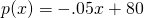. The slope,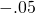, and the intercept,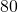, were based on observations by the retailer. The objective of this lesson is to explore methods for finding these numerical values based on a few previous observations. The accepted method for doing this will be explored in more depth later in this course; however, we will occasionally in this lesson assume that the intercept is known, and this will simplify the problem. Assume that the initial price of a suit is $80. When x = 10, 20, and 30 suits have been sold, the price per suit dropped to$79, $77 and$76, respectively. From these observations, we would like to be able to predict the price of a suit for larger x. This will affect the revenue and the profit. If the price drops too low, the profit may be negative!

# The Mathematical Model

The first thing to do is plot the given data. Since we have just four numerical data points (rather than a formula), the best thing to do is put them into an Excel spreadsheet like:

 x (sales) p (market price) 0 80 10 79 20 77 30 76

It's always a good idea to get a look at the data, without any preconceptions. To do this, select the data in the cells, and insert a scatter plot:This will generate a plot of the four data points:This makes it look rather like the data lie on a line. So we guess that the price should be a linear function of the number of suits produced and sold: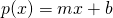There's another, perhaps more pressing reason for this: linear functions are easy to work with and understand! Very often, mathematical modeling involves striking a balance between choosing a mathematical representation that makes sense for the phenomenon we are trying to model, and choosing one that we find easy to work with. This is a version of the modeling approach we saw last time: start with an easy, straightforward model at first and fix it later if we need to.

Back to our particular problem. We know that the intercept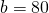, so our quest is to find the slope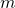.

# Some Mathematical Methods

### The Built-In Solution

screencast: using Excel to compute a least-squares line and the sum-of-squares

The problem of finding the line which best fits some data is such a common thing to do that almost all software for dealing with data has a built-in function for doing in. This includes Excel, most graphing calculators, and a number of websites just a short Google search away.

As we mentioned before, your eye is a pretty good judge of things. We can tell, for example, that the red line in the chart below is a worse fit for the plotted data than the blue line is (actually the red line is a terrible fit for the data points!). But how do we compare the blue line and the green line?Let's see what Excel says. To do this, select your plot. Then click on one of the data points. Then right-click (or option-click on a Mac) and select "Add Trendline..."In the dialog that appears, select "Display equation on chart" and "Display R-squared on chart". You'll notice that two equations,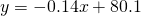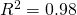appear next to a line on top of the data.

What's going on here, mathematically?

### Correlation

The same thing that your eye did. First Excel checked how linear the data looked. There's a standard mathematical measure of this, called the correlation coefficient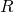. Usually what's reported is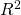; values ofclose to 1 mean the data in question are very close to lying along some line and values ofclose to 0 mean the data do not lie along any particular line. In this case,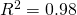means the data are in fact very close to lying along a line. (The formula foris complicated, so it's a good thing to push off onto Excel; we won't say much more about it.)

### What is Least Squares

As for how Excel found which line to draw, that's an interesting story. What Excel is computing is called the least squares line. Let's try to understand where it comes from.

Let's label our datapoints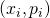, and the model we're trying to build. At this point, we don't know whatand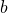are. If our model were perfect, then we'd have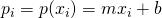so the quantity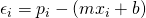measures how far off the model is at the input value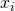. If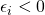, then the model overestimated the data; if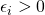then the model underestimated the data. Let's demonstrate this with an example.

Say we picked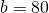and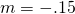as a guess. Then we would populate an Excel sheet as follows:

1. We already have our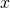and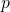data in columns A and B, respectively, but let's make sure everything is labeled; highlight the cells and drag everything down one row so there's a blank row at the top to label 'x' and 'p'.
2. Over in column G (or just someplace off to the right), place a label 'm' and next to it, the label 'b'. Enter '-.15' under 'm' and '80' under 'b'.
3. In column C, place the label 'p(x)' in the first row and the formula '=G$2*A2+H$2' in the second. This is the Excel version of 'p(x)=mx+b'.
4. In column D, place the label 'epsilon' in the first row, and the formula '=B2-C2' in the second. This is the Excel version of '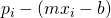'.
5. So far, your table should look like this:6. Now highlight cells C2:D2, copy, and paste into C3:D5. You should obtain:7. Now we can manipulate the values of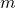and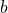we try. For example, try something like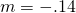or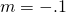, and see what the effect is on the various values of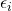.

The formula =G$2*A2 \ H$2, when copied to row 3, becomes =G$2*A3 \ H$2. Any cell name in a formula with a $is absolute: it does not change when moved or copied. Cell names without a$ are relative: when moved or copied, they change by the same amount.

We would like to choose bothandto make the total amount of deviation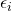as small as possible. Observe that sometimesand sometimes. It could be that a large positivewould cancel a large negative, so simply adding up all thewon't be a good measure of this. Instead, we compute the sum of squares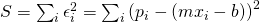It's easy to get Excel to compute the sum of squares

1. Make a column labeled "epsilon-squared" in column E.
2. Enter the formula '=D2^2' in row 2 of this column.
3. Copy that formula into the rest of the column.
4. At the end of the column, in cell E7, sum up the squares by entering '=SUM(E2:E5)'. This is the sum of squares.

Now, try again various values ofand. Watch how the sum of squares changes, to see if you can stumble upon the smallest value of.

### Using Calculus to Find the Least Squares Line

Now, the line Excel reported (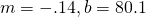) wasn't obtained by guessing and checking. In fact it was found using rather simple calculus.

Recall that the interceptrepresents the price of a suit before we've sold anything -- whatever Excel says, we know we should use, and only worry about finding. Then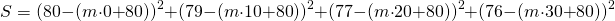is a function of, and easily differentiated: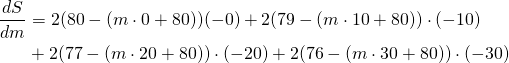We can simplify this a bit: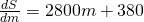and, recalling from Calculus I that to optimize a function we solve the equation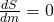, we should solve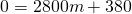to obtain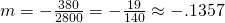This shows that, among all lines with intercept, the one that best fits the data has slope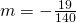.

### Have Maple Do the Hard Part

I've glossed over the calculus bit, and algebra bit, but that's because Maple will do it for us. Just tell it about:

[> S(m):= (80-(m * 0+80))^2+(79-(m * 10+80))^2+(77-(m * 20+80))^2+(76-(m * 30+80))^2

and then differentiate:

[> dS(m):= diff(S(m),m)

and then solve:

[> solve(dS(m)=0,m)

Much easier than doing it by hand.

# Projecting from Data

There are two things we can do with our linear model: we can interpolate, or estimate in between known data points, or extrapolate, that is, attempt to extend beyond the known data. This is also called projecting. For example, we could interpolate the price if we make and sellsuits, or project the price if we make and sell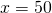suits.

In general, projection puts us on shakier ground than when we interpolate. In this example, we can see quite clearly, that if we extend our linear model far enough, it predicts negative market prices -- which seems unrealistic to say the least!

# Takeaways/Deliverables

To say you've successfully completed this lesson, you should be able to do the following:

1. Use Excel to generate a scatter plot from given data.
2. Use Excel to plot a best-fit line and report its equation.
3. Use Excel to report the correlation coefficient of given data.
4. Use Excel to compute the sum-of-squares measure to see how well a given linear model fits given data.
5. Use Maple to handle the calculus of finding the least-squares line.

These skills are what you'll need to complete the WebAssign homework for this Lesson.